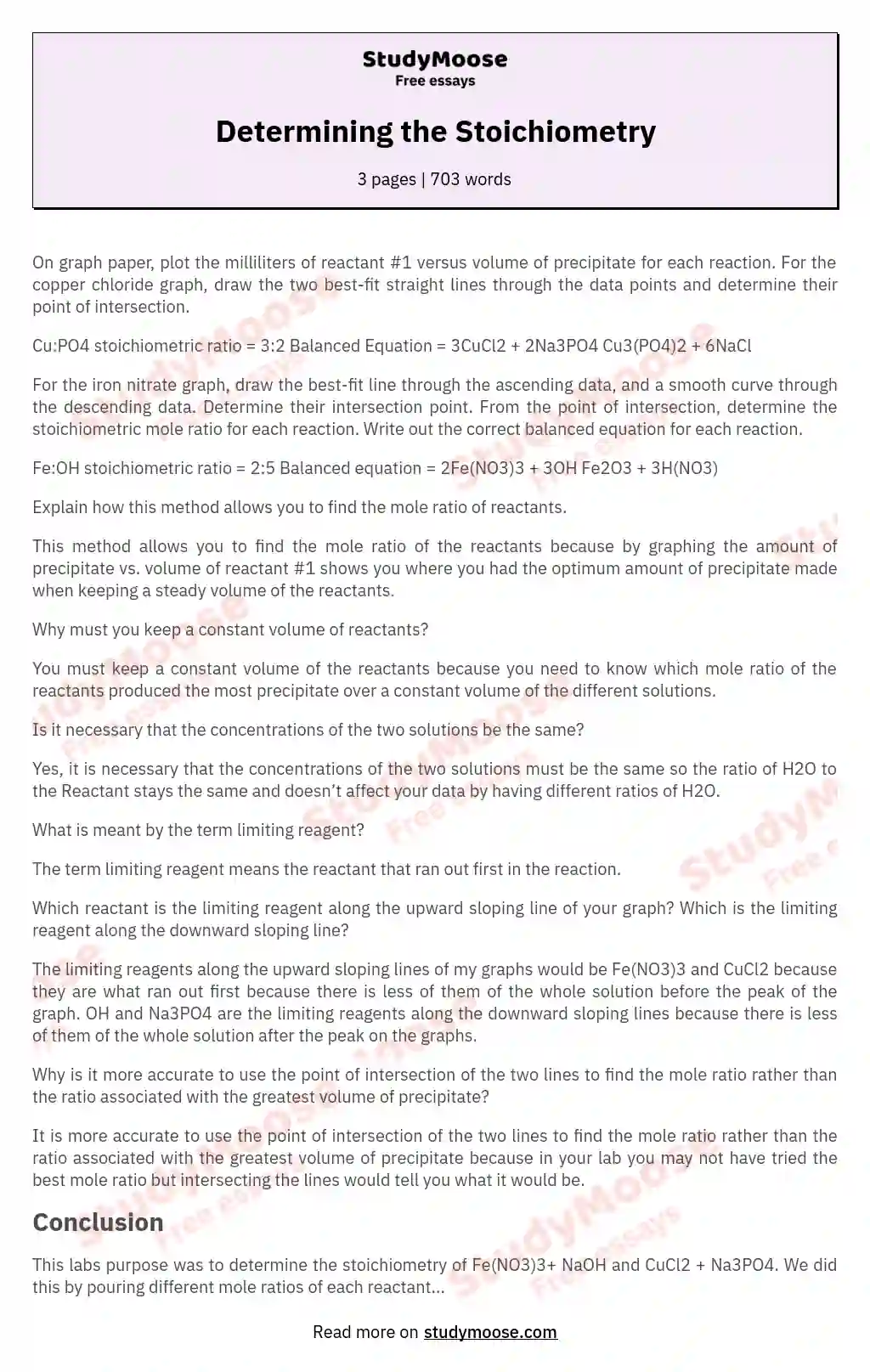# Determining the Stoichiometry

On graph paper, plot the milliliters of reactant #1 versus volume of precipitate for each reaction.

For the copper chloride graph, draw the two best-fit straight lines through the data points and determine their point of intersection.

Cu:PO4 stoichiometric ratio = 3:2
Balanced Equation = 3CuCl2 + 2Na3PO4 Cu3(PO4)2 + 6NaCl

For the iron nitrate graph, draw the best-fit line through the ascending data, and a smooth curve through the descending data. Determine their intersection point. From the point of intersection, determine the stoichiometric mole ratio for each reaction. Write out the correct balanced equation for each reaction.

Fe:OH stoichiometric ratio = 2:5
Balanced equation = 2Fe(NO3)3 + 3OH Fe2O3 + 3H(NO3)

Explain how this method allows you to find the mole ratio of reactants.

This method allows you to find the mole ratio of the reactants because by graphing the amount of precipitate vs. volume of reactant #1 shows you where you had the optimum amount of precipitate made when keeping a steady volume of the reactants.

Get quality help nowwriter-marianVerified writer

Proficient in: Chemistry Stoichiometry4.8 (309)

“ Writer-marian did a very good job with my paper, she got straight to the point, she made it clear and organized ”+84 relevant experts are online

Why must you keep a constant volume of reactants?

You must keep a constant volume of the reactants because you need to know which mole ratio of the reactants produced the most precipitate over a constant volume of the different solutions.

Is it necessary that the concentrations of the two solutions be the same?

Yes, it is necessary that the concentrations of the two solutions must be the same so the ratio of H2O to the Reactant stays the same and doesn’t affect your data by having different ratios of H2O.

Get to Know The Price Estimate For Your Paper
Topic
Number of pages
Email Invalid email

You won’t be charged yet!

What is meant by the term limiting reagent?

The term limiting reagent means the reactant that ran out first in the reaction.

Which reactant is the limiting reagent along the upward sloping line of your graph? Which is the limiting reagent along the downward sloping line?

The limiting reagents along the upward sloping lines of my graphs would be Fe(NO3)3 and CuCl2 because they are what ran out first because there is less of them of the whole solution before the peak of the graph. OH and Na3PO4 are the limiting reagents along the downward sloping lines because there is less of them of the whole solution after the peak on the graphs.

Why is it more accurate to use the point of intersection of the two lines to find the mole ratio rather than the ratio associated with the greatest volume of precipitate?

It is more accurate to use the point of intersection of the two lines to find the mole ratio rather than the ratio associated with the greatest volume of precipitate because in your lab you may not have tried the best mole ratio but intersecting the lines would tell you what it would be.

## Conclusion

This labs purpose was to determine the stoichiometry of Fe(NO3)3+ NaOH and CuCl2 + Na3PO4. We did this by pouring different mole ratios of each reactant to reactant in 7 different graduated cylinders all consisting of the same volume. Then we measured the volume of precipitate of each cylinder and graphed volume of reactant #1 vs. volume of precipitate for each reaction. In our graphs it showed us the rising up to a peak the going back down. I, then drew the line of best fit threw the ascending data and descending data, and were the lines intersect would be our optimum ratio.

For these particular reactions our max point was our optimum mole ratios, If we did not mix that max ratio it wouldn’t be on our graph so that is why I used lines of best fit because it would show you were your optimum mole ratio would be. In my Fe(NO3)3 + NaOH graph/data it shows another jump back up from cylinder 6 to cylinder 7 which does not make sense the descending data should not jump back up. Some possible sources of error that might have caused this could be someone getting cylinders 6 and 7 mixed up or not measuring the right amounts of solutions to be mixed together. After doing all this I was able to determine the balanced equations 2Fe(NO3)3 + 3OH Fe2O3 + 3H(NO3) and 3CuCl2 + 2Na3PO4 Cu3(PO4)2 + 6NaCl.